# 简介：Compose 中的状态

## 1. 准备工作

• 在无法建立网络连接时显示的消息。
• 表单，例如注册表单。状态可以填写和提交。
• 可点按的控件，例如按钮。状态可以是“未点按”“正在点按”（显示动画）或“已点按”（一种 `onClick` 操作）。

• `TextField` 可组合项，用于输入和修改文本。
• `Text` 可组合项，用于显示文本。
• `Spacer` 可组合项，用于显示界面元素之间的空白空间。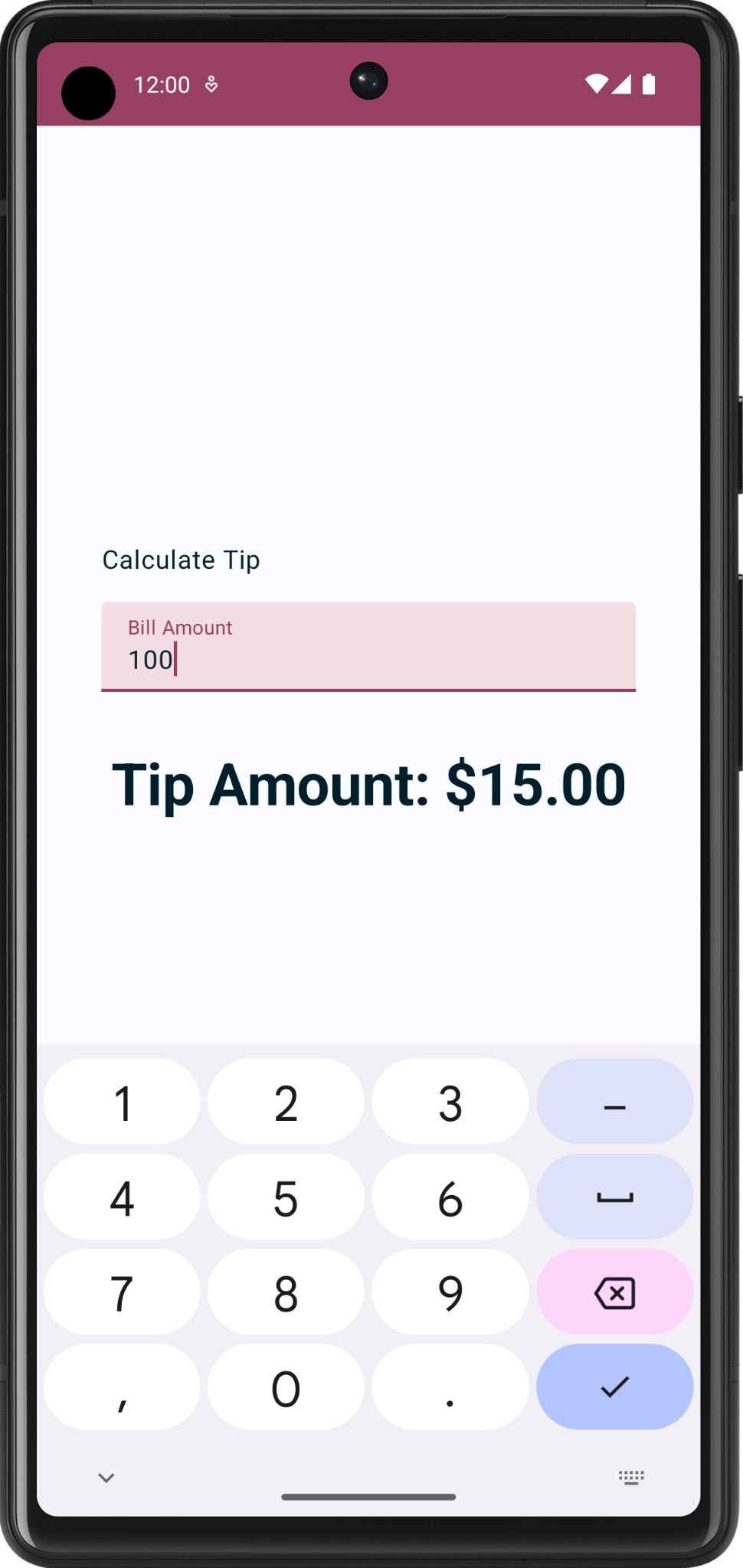## 前提条件

• 对 Compose（如 `@Composable` 注解）有基本的了解。
• 基本熟悉 Compose 布局，例如 `Row``Column` 布局可组合项。
• 基本熟悉修饰符，例如 `Modifier.padding()` 函数。
• 熟悉 `Text` 可组合项。

## 学习内容

• 如何看待界面中的状态。
• Compose 如何使用状态显示数据。
• 如何向应用添加文本框。
• 如何提升状态。

## 您将构建的内容

• 一款名为 Tip Time 的小费计算器应用，用于根据服务金额计算小费金额。

## 所需条件

• 可连接到互联网的计算机和网络浏览器
• 了解 Kotlin
• 最新版本的 Android Studio

## 2. 开始

1. 请查看 Google 的在线小费计算器。请注意，这只是一个示例，它不是您将在本课程中创建的 Android 应用。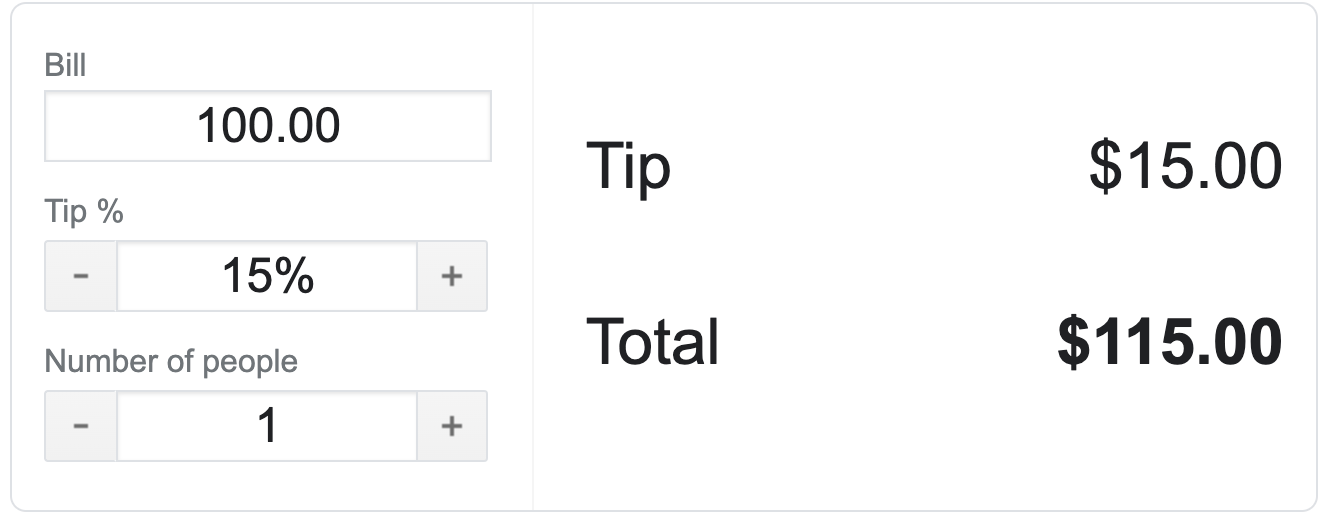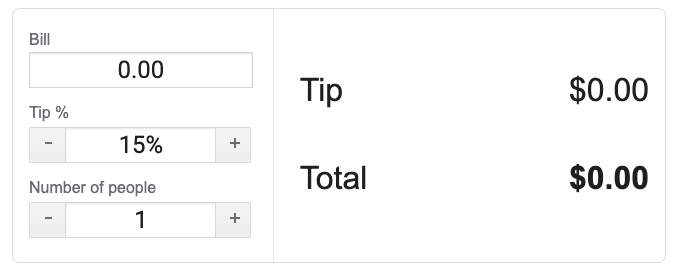1. BillTip % 框中输入不同的值。小费和总金额的值会随之改变。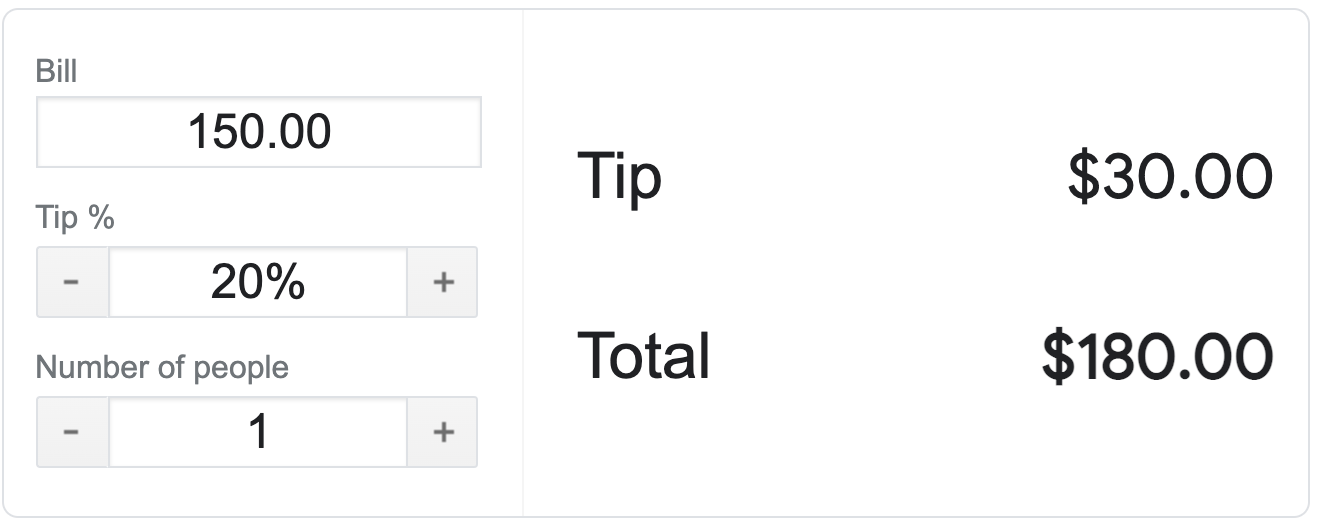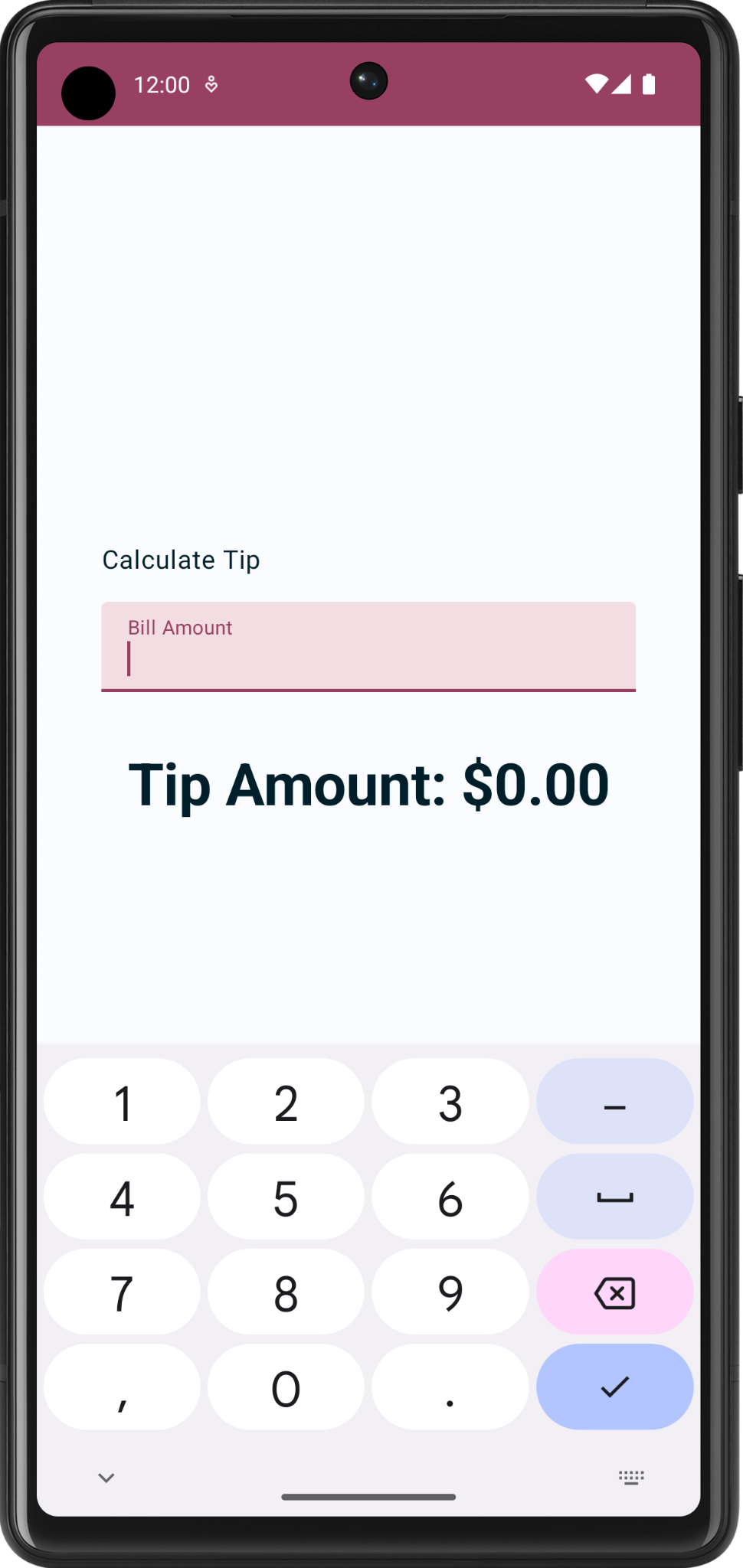## 3. 获取起始代码

```\$ git clone https://github.com/google-developer-training/basic-android-kotlin-compose-training-tip-calculator.git
\$ cd basic-android-kotlin-compose-training-tip-calculator
\$ git checkout starter
```

## 起始应用概览

1. 在 Android Studio 中打开包含起始代码的项目。
2. 在 Android 设备或模拟器上运行该应用。
3. 您会发现两个文本组件；一个用于显示标签，另一个用于显示小费金额。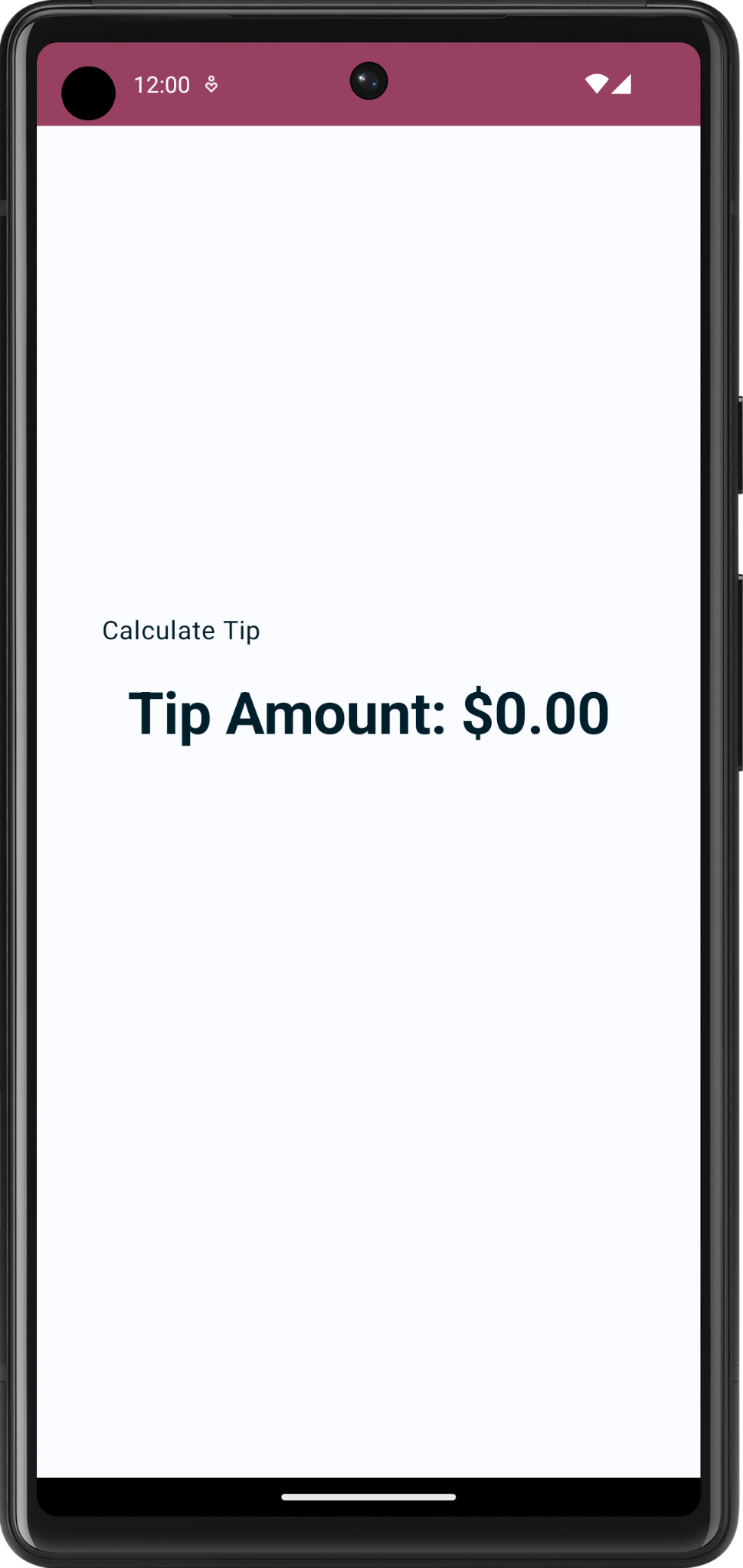1. 请注意，顶部的状态栏颜色与您通常使用 Empty Activity 模板制作的应用中的常见颜色有何不同。出现这种不同的原因在于它使用自定义样式和主题。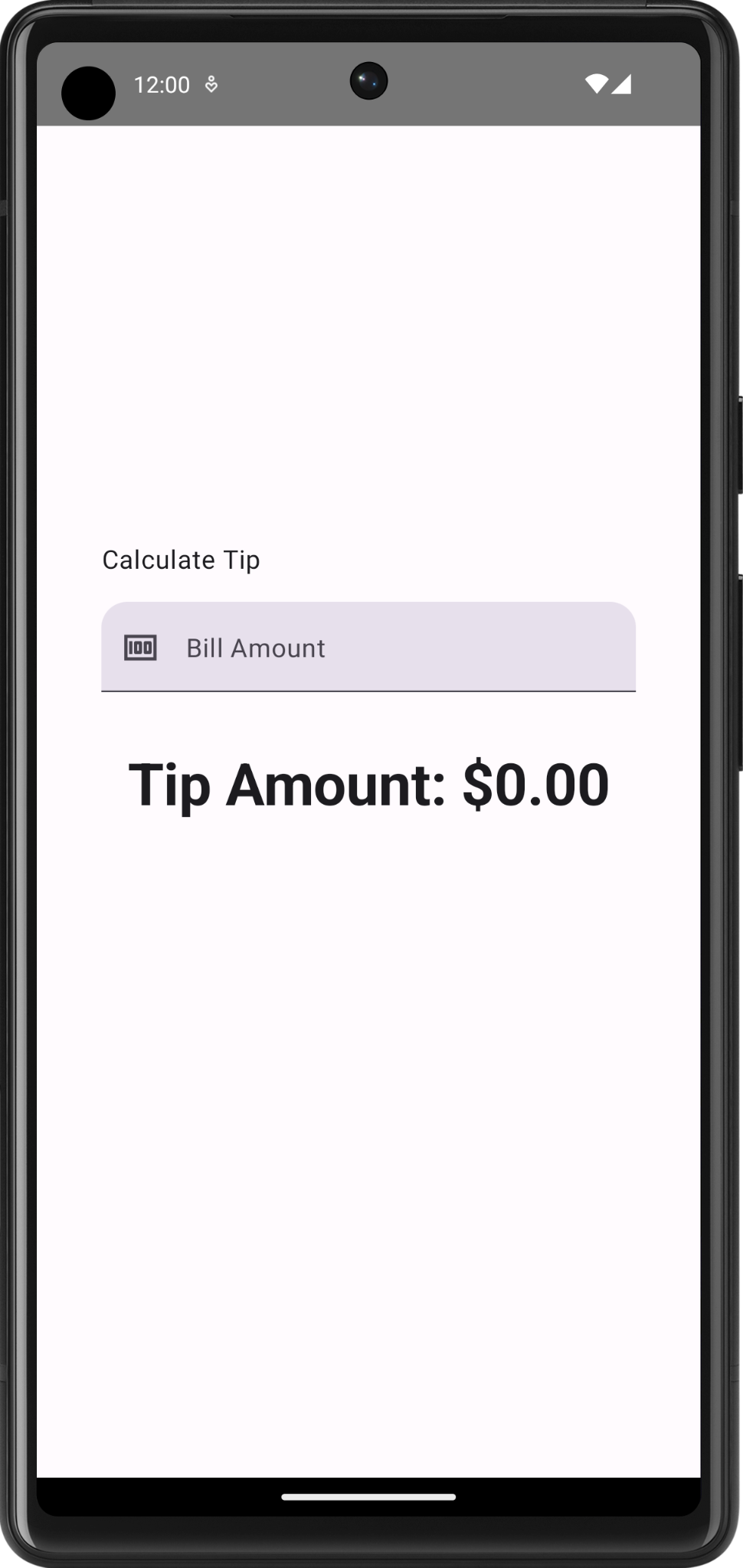未采用自定义主题。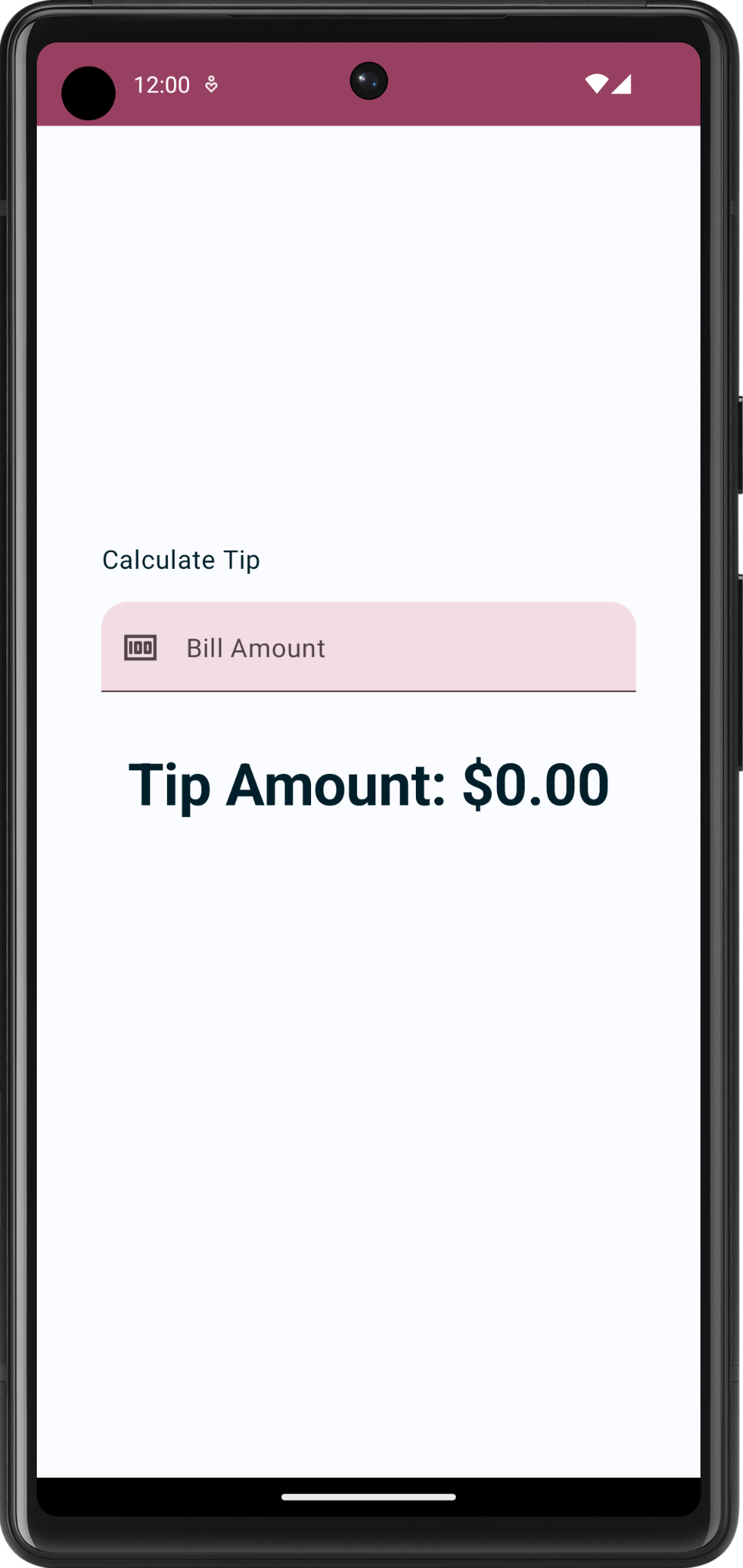采用了自定义主题。

## 起始代码演示

res > values > strings.xml

``````<resources>
<string name="app_name">Tip Time</string>
<string name="calculate_tip">Calculate Tip</string>
<string name="bill_amount">Bill Amount</string>
<string name="tip_amount">Tip Amount: %s</string>
</resources>
``````

MainActivity

• `TipTimeLayout()` 函数包含一个 `Column` 元素，后者包含您在屏幕截图中看到的两个文本可组合项。这还包含一个 `spacer` 可组合项，以出于美观原因而添加空间。
• `calculateTip()` 函数，用于接受账单金额并按 15% 的百分比来计算小费金额。`tipPercent` 形参设置为一个 `15.0` 默认实参值。这会暂时将默认小费百分比值设置为 15%。在下一个 Codelab 中，您将从用户那获取小费金额。
``````@Composable
fun TipTimeLayout() {
Column(
horizontalAlignment = Alignment.CenterHorizontally,
verticalArrangement = Arrangement.Center
) {
Text(
text = stringResource(R.string.calculate_tip),
modifier = Modifier
.align(alignment = Alignment.Start)
)
Text(
text = stringResource(R.string.tip_amount, "\$0.00"),
style = MaterialTheme.typography.displaySmall
)
Spacer(modifier = Modifier.height(150.dp))
}
}
``````
``````private fun calculateTip(amount: Double, tipPercent: Double = 15.0): String {
val tip = tipPercent / 100 * amount
return NumberFormat.getCurrencyInstance().format(tip)
}
``````

`onCreate()` 函数的 `Surface()` 代码块中，调用 `TipTimeLayout()` 函数。这样便可在设备或模拟器中显示该应用的布局。

``````override fun onCreate(savedInstanceState: Bundle?) {
//...
setContent {
TipTimeTheme {
Surface(
//...
) {
TipTimeLayout()
}
}
}
}
``````

`TipTimeLayoutPreview()` 函数的 `TipTimeTheme` 代码块中，调用 `TipTimeLayout()` 函数。这样，在 DesignSplit 窗格中便会显示该应用的布局。

``````@Preview(showBackground = true)
@Composable
fun TipTimeLayoutPreview() {
TipTimeTheme {
TipTimeLayout()
}
}
``````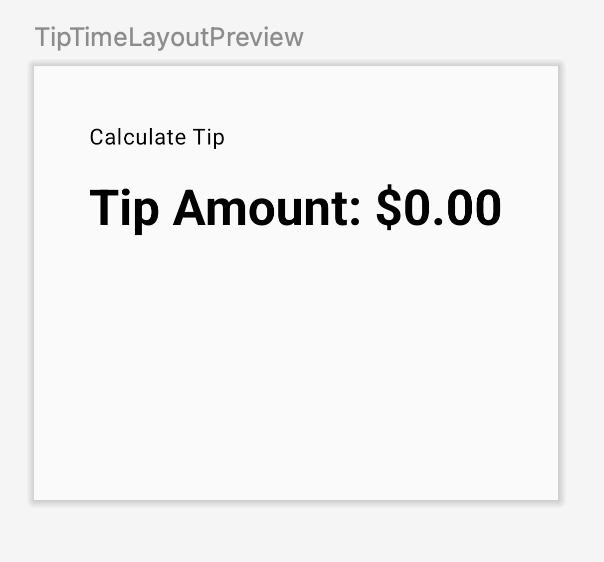## 接受用户的输入内容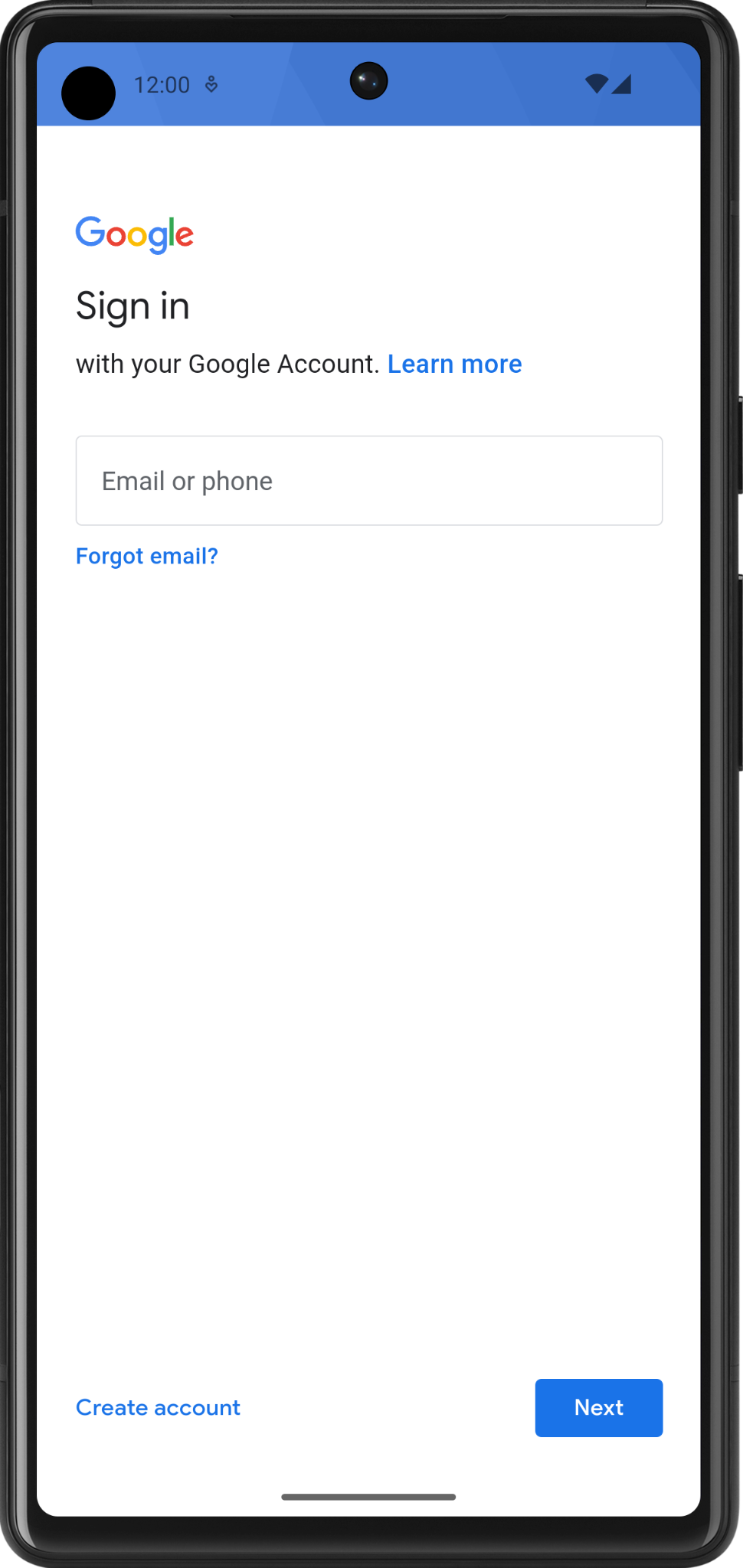`TextField` 可组合项添加到应用中：

1. `MainActivity.kt` 文件中，添加一个接受 `Modifier` 形参的 `EditNumberField()` 可组合函数。
2. `TipTimeLayout()` 下的 `EditNumberField()` 函数的正文中，添加一个 `TextField`，用于接受设置为空字符串的 `value` 具名形参以及一个设置为空 lambda 表达式的 `onValueChange` 具名形参：
``````@Composable
fun EditNumberField(modifier: Modifier = Modifier) {
TextField(
value = "",
onValueChange = {},
modifier = modifier
)
}
``````
1. 请注意您传递的形参：
• `value` 形参是一个文本框，用于显示您在此处传递的字符串值。
• `onValueChange` 形参是用户在文本框中输入文本时触发的 lambda 回调。
1. 导入以下函数：
``````import androidx.compose.material3.TextField
``````
1. `TipTimeLayout()` 可组合项中第一个文本可组合函数后面的代码行中，调用 `EditNumberField()` 函数，并传递以下修饰符。
``````import androidx.compose.foundation.layout.fillMaxWidth

@Composable
fun TipTimeLayout() {
Column(
horizontalAlignment = Alignment.CenterHorizontally,
verticalArrangement = Arrangement.Center
) {
Text(
...
)
Text(
...
)
...
}
}
``````

1. Design 窗格中，您应该会看到 `Calculate Tip` 文本、一个空文本框和 `Tip Amount` 文本可组合项。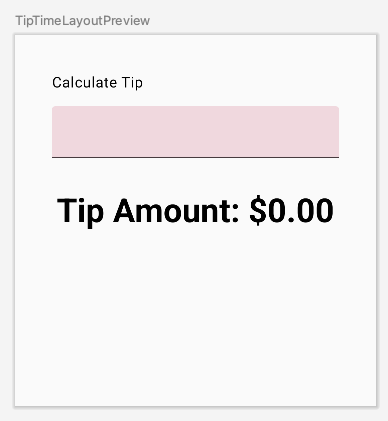## 4. 在 Compose 中使用状态

1. `EditNumberField()` 函数的开头，使用 `val` 关键字添加 `amountInput` 变量，并将它设为 `"0"` 值：
``````val amountInput = "0"
``````

1. `value` 具名形参设置为 `amountInput` 值：
``````TextField(
value = amountInput,
onValueChange = {},
)
``````
1. 检查预览效果。文本框会显示设置为状态变量的值，如下图所示：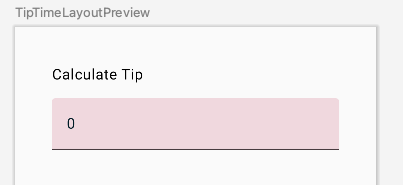1. 在模拟器中运行该应用，尝试输入其他值。硬编码状态保持不变，因为 `TextField` 可组合项不会自行更新。当 `value` 形参（设置为 `amountInput` 属性）更改时，它会更新。

`amountInput` 变量表示文本框的状态。具有硬编码状态并没有什么用处，因为它无法修改，也无法反映用户输入。您需要在用户更新账单金额时更新应用状态。

## 5. 组合

Compose 是一个声明性界面框架，这意味着您可以在代码中声明界面的外观。如果您想让文本框一开始显示 `100` 值，请在代码中将可组合项的初始值设置为 `100` 值。

“组合”是 Compose 在执行可组合项时构建的界面描述。Compose 应用调用可组合函数，以将数据转换为界面。如果发生状态更改，Compose 会使用新状态重新执行受影响的可组合函数，从而创建更新后的界面。这一过程称为“重组”。Compose 会为您安排重组。

`mutableStateOf()` 函数返回的值：

• 会保持状态，即账单金额。
• 可变，因此该值可以更改。
• 可观察，因此 Compose 会观察值的所有更改并触发重组以更新界面。

1. `EditNumberField()` 函数中，将 `amountInput` 状态变量前面的 `val` 关键字更改为 `var` 关键字：
``````var amountInput = "0"
``````

1. 使用 `MutableState<String>` 类型（而非硬编码的 `String` 变量），以便 Compose 知道要跟踪 `amountInput` 状态，然后传入 `"0"` 字符串，该字符串是 `amountInput` 状态变量的初始默认值：
``````import androidx.compose.runtime.MutableState
import androidx.compose.runtime.mutableStateOf

var amountInput: MutableState<String> = mutableStateOf("0")
``````

``````var amountInput = mutableStateOf("0")
``````

`mutableStateOf()` 函数接受初始值 `"0"` 作为实参，这样便可使其 `amountInput` 变为可观察。这会导致 Android Studio 中出现以下编译警告，但您很快就可以修复此问题：

```Creating a state object during composition without using remember.
```
1. `TextField` 可组合函数中，使用 `amountInput.value` 属性：
``````TextField(
value = amountInput.value,
onValueChange = {},
modifier = modifier
)
``````

Compose 会跟踪每个读取状态 `value` 属性的可组合项，并在其 `value` 更改时触发重组。

1. `onValueChange` 具名形参的 lambda 表达式中，将 `amountInput.value` 属性设置为 `it` 变量：
``````@Composable
fun EditNumberField(modifier: Modifier = Modifier) {
var amountInput = mutableStateOf("0")
TextField(
value = amountInput.value,
onValueChange = { amountInput.value = it },
modifier = modifier
)
}
``````

`TextField` 通过 `onValueChange` 回调函数通知您文本发生更改时，您将更新 `TextField` 的状态（即 `amountInput` 变量）。

1. 运行应用并在文本框中输入文本。文本框仍会显示 `0` 值，如下图所示：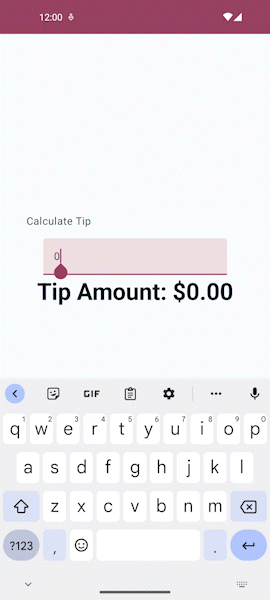## 6. 使用 remember 函数保存状态

`EditNumberField()` 函数中使用 `remember` 函数：

1. `EditNumberField()` 函数中，使用 `remember` 将对 `mutableStateOf``()` 的调用括起来，以便使用 `by` `remember` Kotlin 属性委托来初始化 `amountInput` 变量。
2. `mutableStateOf``()` 函数中，传入一个空字符串（而非静态 `"0"` 字符串）：
``````var amountInput by remember { mutableStateOf("") }
``````

1. 导入以下函数：
``````import androidx.compose.runtime.remember
import androidx.compose.runtime.getValue
import androidx.compose.runtime.setValue
``````

``````@Composable
fun EditNumberField(modifier: Modifier = Modifier) {
var amountInput by remember { mutableStateOf("") }
TextField(
value = amountInput,
onValueChange = { amountInput = it },
modifier = modifier
)
}
``````
1. 运行应用并在文本框中输入一些文本。现在，您应该会看到您输入的文本。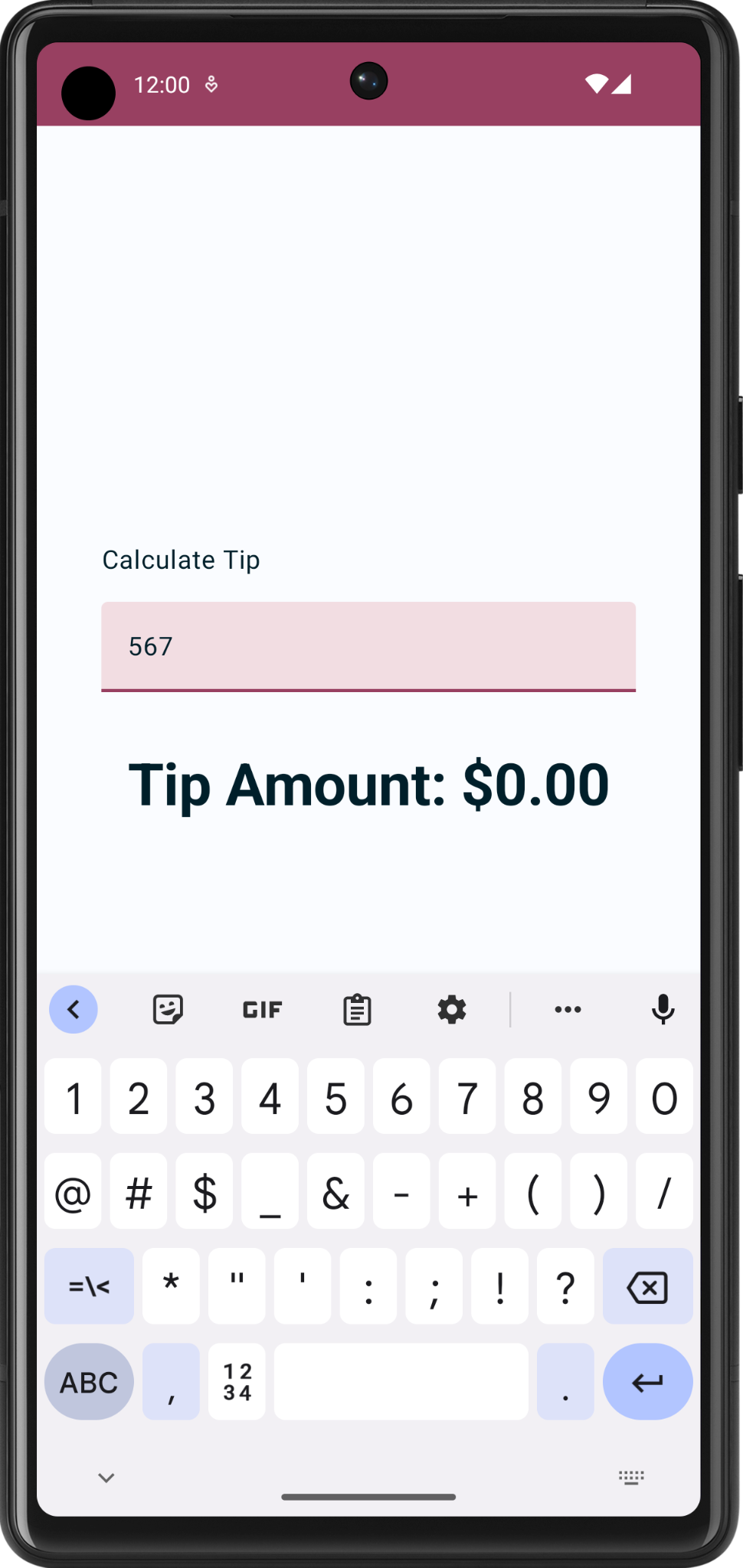## 7. 状态和重组的实际运用

1. `onValueChange` 具名函数旁边的 `EditNumberField()` 函数中，设置一个行断点。
2. 在导航菜单中，点击 Debug 'app' 图标。应用会在模拟器或设备上启动。创建 `TextField` 元素后，应用执行会第一次暂停。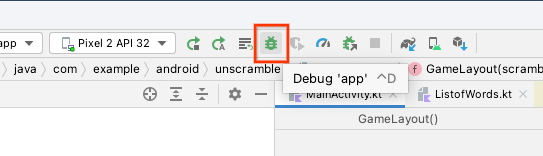1. Debug 窗格中，点击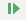Resume Program。文本框创建完毕。
2. 在模拟器或设备上的文本框中输入一个字母。当应用到达您设置的断点时，应用执行会再次暂停。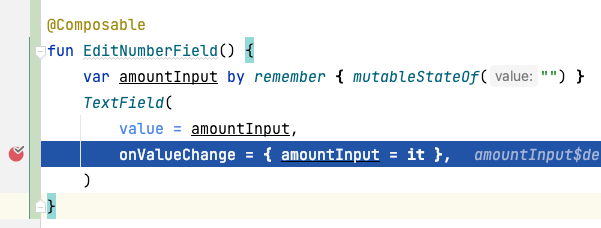1. Debug 窗格中，点击Resume Program。在模拟器或设备上输入的文本会显示在包含断点的代码行旁边，如下图所示：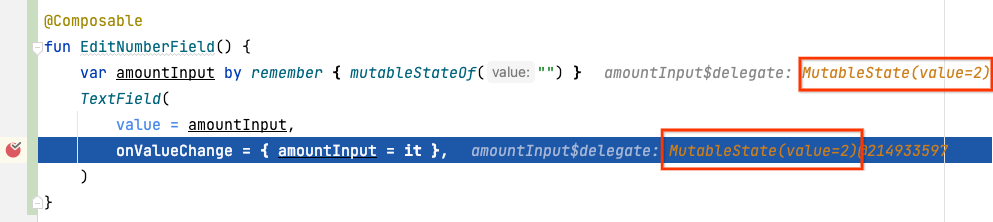1. 点击Resume Program。输入的值会显示在模拟器或设备上。

## 向文本框添加标签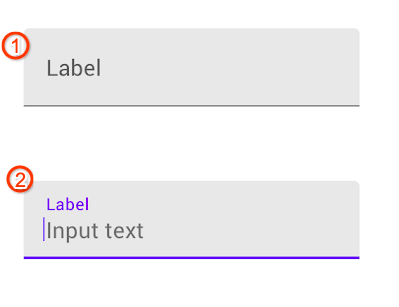1. `EditNumberField()` 函数的 `TextField()` 可组合函数中，添加一个设置为空 lambda 表达式的 `label` 具名形参：
``````TextField(
//...
label = { }
)
``````
1. 在 lambda 表达式中，调用接受 `stringResource``(R.string.``bill_amount``)``Text()` 函数：
``````label = { Text(stringResource(R.string.bill_amount)) },
``````
1. `TextField()` 可组合函数中，添加设置为 `true` 值的 `singleLine` 具名形参：
``````TextField(
// ...
singleLine = true,
)
``````

1. 添加设置为 `KeyboardOptions()``keyboardOptions` 形参：
``````import androidx.compose.foundation.text.KeyboardOptions

TextField(
// ...
keyboardOptions = KeyboardOptions(),
)
``````

Android 提供了一个选项，用于配置屏幕上显示的键盘，以便输入数字、电子邮件地址、网址和密码等内容。如需详细了解其他键盘类型，请参阅 KeyboardType

1. 将键盘类型设置为数字键盘即可输入数字。向 `KeyboardOptions` 函数传递设置为 `KeyboardType.Number``keyboardType` 具名形参：
``````import androidx.compose.ui.text.input.KeyboardType

TextField(
// ...
keyboardOptions = KeyboardOptions(keyboardType = KeyboardType.Number),
)
``````

``````@Composable
fun EditNumberField(modifier: Modifier = Modifier) {
var amountInput by remember { mutableStateOf("") }
TextField(
value = amountInput,
onValueChange = { amountInput = it },
singleLine = true,
label = { Text(stringResource(R.string.bill_amount)) },
keyboardOptions = KeyboardOptions(keyboardType = KeyboardType.Number),
modifier = modifier
)
}
``````
1. 运行应用。## 9. 显示小费金额

`MainActivity.kt` 文件中，起始代码中已为您提供了 `private` `calculateTip()` 函数。您将使用此函数计算小费金额：

``````private fun calculateTip(amount: Double, tipPercent: Double = 15.0): String {
val tip = tipPercent / 100 * amount
return NumberFormat.getCurrencyInstance().format(tip)
}
``````

## 使用 `calculateTip()` 函数

1. `EditNumberField()` 可组合函数中，在 `amountInput` 定义之后创建一个名为 `amount` 的新变量。对 `amountInput` 变量调用 `toDoubleOrNull` 函数，以将 `String` 转换为 `Double`
``````val amount = amountInput.toDoubleOrNull()
``````

`toDoubleOrNull()` 函数是一个预定义的 Kotlin 函数，该函数会将字符串解析为 `Double` 数字并返回结果，而如果字符串不是有效的数字表示法，该函数会返回 `null`

1. 在语句末尾，添加一个 `?:` Elvis 运算符，以在 `amountInput` 为 null 时返回 `0.0` 值：
``````val amount = amountInput.toDoubleOrNull() ?: 0.0
``````
1. `amount` 变量后面，再创建一个名为 `tip``val` 变量。使用 `calculateTip()` 对其进行初始化，并传递 `amount` 形参。
``````val tip = calculateTip(amount)
``````

`EditNumberField()` 函数应如以下代码段所示：

``````@Composable
fun EditNumberField(modifier: Modifier = Modifier) {
var amountInput by remember { mutableStateOf("") }

val amount = amountInput.toDoubleOrNull() ?: 0.0
val tip = calculateTip(amount)

TextField(
value = amountInput,
onValueChange = { amountInput = it },
label = { Text(stringResource(R.string.bill_amount)) },
modifier = Modifier.fillMaxWidth(),
singleLine = true,
keyboardOptions = KeyboardOptions(keyboardType = KeyboardType.Number)
)
}
``````

## 显示计算出的小费金额

1. `TipTimeLayout()` 函数中的 `Column()` 代码块的末尾，请注意那个显示 `\$0.00` 的文本可组合项。您将要把此值更新为计算出的小费金额。
``````@Composable
fun TipTimeLayout() {
Column(
horizontalAlignment = Alignment.CenterHorizontally,
verticalArrangement = Arrangement.Center
) {
// ...
Text(
text = stringResource(R.string.tip_amount, "\$0.00"),
style = MaterialTheme.typography.displaySmall
)
// ...
}
}
``````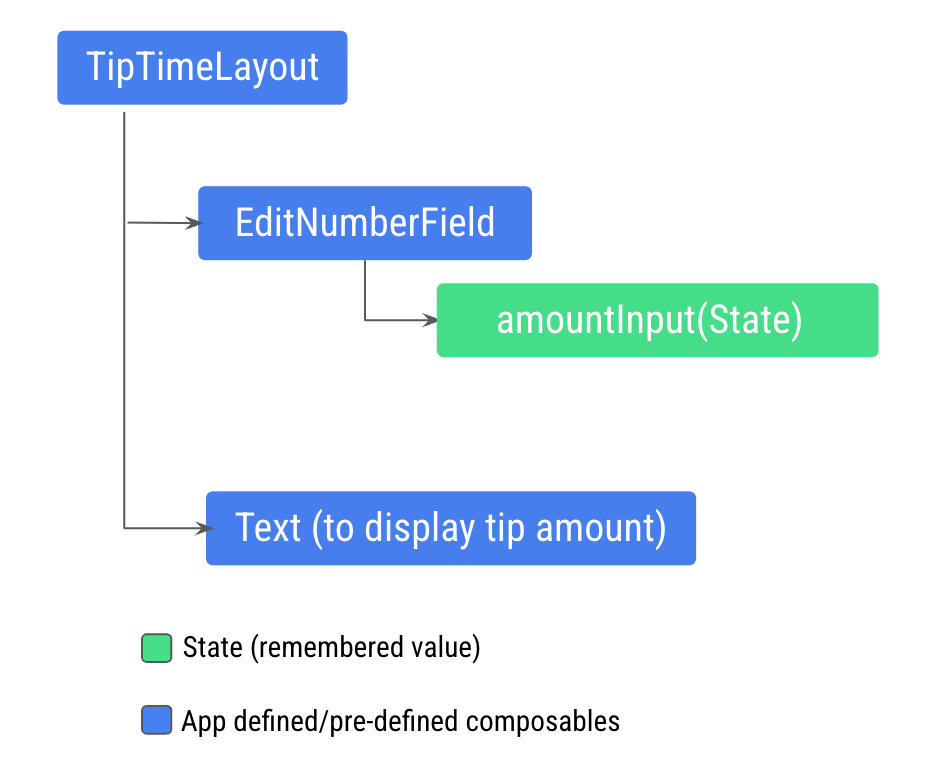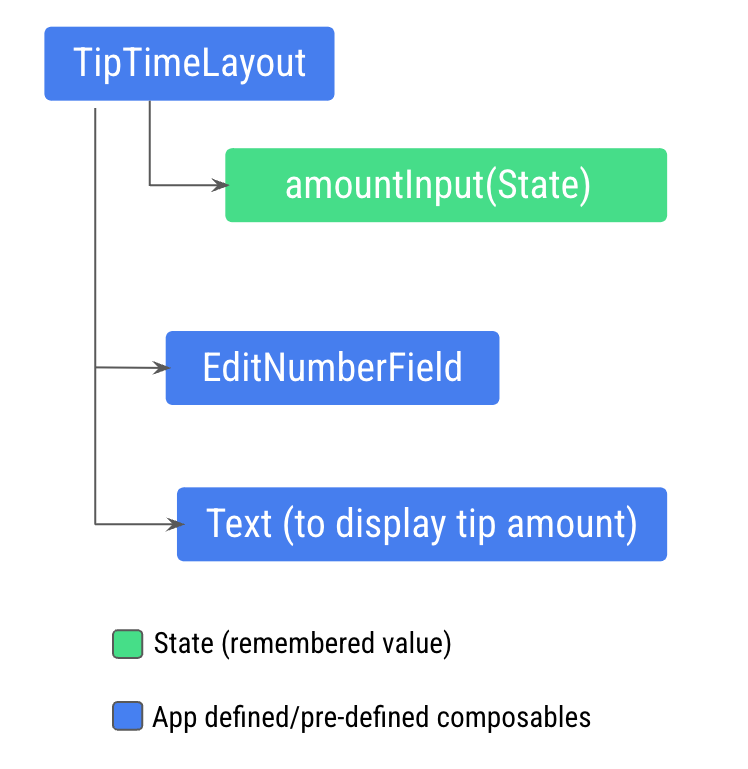## 了解有状态和无状态可组合项

• 与多个可组合函数共享状态。
• 创建可在应用中重复使用的无状态可组合项。

• `value: T` 形参，即要显示的当前值。
• `onValueChange: (T) -> Unit` - 回调 lambda，会在值更改时触发，以便可以在其他位置更新状态（例如，当用户在文本框中输入一些文本时）。

`EditNumberField()` 函数中提升状态：

1. 更新 `EditNumberField()` 函数定义，以通过添加 `value``onValueChange` 形参来提升状态：
``````@Composable
fun EditNumberField(
value: String,
onValueChange: (String) -> Unit,
modifier: Modifier = Modifier
) {
//...
``````

`value` 形参的类型为 `String``onValueChange` 形参的类型为 `(String) -> Unit`，因此它是一个接受 `String` 值作为输入且没有返回值的函数。`onValueChange` 形参用作传入 `TextField` 可组合项的 `onValueChange` 回调。

1. `EditNumberField()` 函数中，更新 `TextField()` 可组合函数以使用传入的形参：
``````TextField(
value = value,
onValueChange = onValueChange,
// Rest of the code
)
``````
1. 提升状态，将记住的状态从 `EditNumberField()` 函数移至 `TipTimeLayout()` 函数：
``````@Composable
fun TipTimeLayout() {
var amountInput by remember { mutableStateOf("") }

val amount = amountInput.toDoubleOrNull() ?: 0.0
val tip = calculateTip(amount)

Column(
//...
) {
//...
}
}
``````
1. 您已将状态提升到 `TipTimeLayout()`，现在将其传递到 `EditNumberField()`。在 `TipTimeLayout()` 函数中，更新 `EditNumberField``()` 函数调用以使用提升的状态：
``````EditNumberField(
value = amountInput,
onValueChange = { amountInput = it },
modifier = Modifier
.fillMaxWidth()
)
``````

## 位置格式设置

``````// No need to copy.

// In the res/values/strings.xml file
<string name="tip_amount">Tip Amount: %s</string>
``````

``````// No need to copy
Text(
text = stringResource(R.string.tip_amount, "\$0.00"),
style = MaterialTheme.typography.displaySmall
)
``````
1. 在函数 `TipTimeLayout()` 中，使用 `tip` 属性显示小费金额。更新 `Text` 可组合项的 `text` 形参，以将 `tip` 变量用作形参。
``````Text(
text = stringResource(R.string.tip_amount, tip),
// ...
``````

``````@Composable
fun TipTimeLayout() {
var amountInput by remember { mutableStateOf("") }
val amount = amountInput.toDoubleOrNull() ?: 0.0
val tip = calculateTip(amount)

Column(
horizontalAlignment = Alignment.CenterHorizontally,
verticalArrangement = Arrangement.Center
) {
Text(
text = stringResource(R.string.calculate_tip),
modifier = Modifier
.align(alignment = Alignment.Start)
)
EditNumberField(
value = amountInput,
onValueChange = { amountInput = it },
modifier = Modifier
.fillMaxWidth()
)
Text(
text = stringResource(R.string.tip_amount, tip),
style = MaterialTheme.typography.displaySmall
)
Spacer(modifier = Modifier.height(150.dp))
}
}

@Composable
fun EditNumberField(
value: String,
onValueChange: (String) -> Unit,
modifier: Modifier = Modifier
) {
TextField(
value = value,
onValueChange = onValueChange,
singleLine = true,
label = { Text(stringResource(R.string.bill_amount)) },
keyboardOptions = KeyboardOptions(keyboardType = KeyboardType.Number),
modifier = modifier
)
}
``````

1. 在模拟器或设备上运行应用，然后在 Bill Amount 文本框中输入一个值。系统即会显示小费金额（账单金额的 15%），如下图所示：## 11. 获取解决方案代码

```\$ git clone https://github.com/google-developer-training/basic-android-kotlin-compose-training-tip-calculator.git
\$ cd basic-android-kotlin-compose-training-tip-calculator
\$ git checkout state
```

## 总结

• 应用中的状态是指可以随时间变化的任何值。
• “组合”是 Compose 在执行可组合项时构建的界面描述。Compose 应用调用可组合函数，以将数据转换为界面。
• 初始组合是指 Compose 会在首次执行可组合函数时创建界面。
• 重组过程会再次运行相同的可组合项，以便在其数据发生变化时更新树。
• 状态提升是一种将状态移到其调用方以使组件变为无状态的模式。

[]
[]